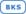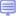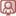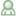Training Console
877-727-4678

# SAS/ETS

Title Level Training Formats
Establishing Causal Inferences: Propensity Score Matching, Heckman's Two-Stage Model, Interrupted Time Series, and Regression Discontinuity ModelsThis course introduces some methods commonly used in program evaluation and real-world effectiveness studies, including two-stage modeling, interrupted time-series, regression discontinuity, and propensity score matching. These methods help address questions such as: Which medicine is more effective in the real world? Did an advertising program have an impact on sales? More generally, are the changes in outcomes causally related to the program being run?

3 IntermediateStationarity Testing and Other Time Series TopicsThis course addresses a basic question in time series modeling and forecasting: whether a time series is nonstationary. This question is addressed by the unit root tests. One of the most common tests, the Dickey-Fuller test, is discussed in this lecture.

3 IntermediateUsing SAS Forecast Server Procedures
This course teaches you how to create and manage a complete forecasting system using the SAS Forecast Server procedures, giving you the power to confidently plan your business operations.

3 IntermediateModels for Time Series and Sequential Data
This course teaches students to build, refine, extrapolate, and, in some cases, interpret models designed for a single, sequential series. There are three modeling approaches presented. The traditional, Box-Jenkins approach for modeling time series is covered in the first part of the course. This presentation moves students from models for stationary data (or ARMA) to models for trend and seasonality (ARIMA) and concludes with information about specifying transfer function components in an ARIMAX, or time series regression, model. A Bayesian approach to modeling time series is considered next. The basic Bayesian framework is extended to accommodate autoregressive variation in the data as well as dynamic input variable effects. Machine learning algorithms for time series is the third approach. Gradient boosting and recurrent neural network algorithms are particularly well suited for accommodating nonlinear relationships in the data. Examples are provided to build intuition on the effective use of these algorithms. The course concludes by considering how forecasting precision can be improved by combining the strengths of the different approaches. The final lesson includes demonstrations of creating combined (or ensemble) and hybrid model forecasts.

3 IntermediateStatistics 2: ANOVA and Regression
This course teaches you how to analyze continuous response data and discrete count data. Linear regression, Poisson regression, negative binomial regression, gamma regression, analysis of variance, linear regression with indicator variables, analysis of covariance, and mixed models ANOVA are presented in the course.

3 IntermediateTime Series Modeling Essentials
This course discusses the fundamentals of modeling time series data. The course focuses on the applied use of the three main model types used to analyze univariate time series: exponential smoothing, autoregressive integrated moving average with exogenous variables (ARIMAX), and unobserved components (UCM).

The e-learning format of this course includes Virtual Lab time to practice.

3 IntermediateTime Series Feature Mining and Creation
In this course, you learn about data exploration, feature creation, and feature selection for time sequences. The topics discussed include binning, smoothing, transformations, and data set operations for time series, spectral analysis, singular spectrum analysis, distance measures, and motif analysis.

3 IntermediateForecasting Using SAS Software: A Programming Approach
This course teaches analysts how to use SAS/ETS software to diagnose systematic variation in data collected over time, create forecast models to capture the systematic variation, evaluate a given forecast model for goodness of fit and accuracy, and forecast future values using the model. Topics include Box-Jenkins ARIMA models, dynamic regression models, and exponential smoothing models.

4 ExpertState Space Modeling Essentials Using the SSM Procedure in SAS/ETS
This course covers the fundamentals of building and applying state space models using the SSM procedure (SAS/ETS). Students are presented with an overview of the model and learn advantages of the State Space approach. The course also describes fundamental model details, presents some straightforward examples of specifying and fitting models using the SSM procedure, and considers estimation in SSM, focusing on the Kalman filter and related details. The course concludes with a variety of SSM modeling applications, focused mainly on time series.

4 Expert